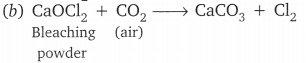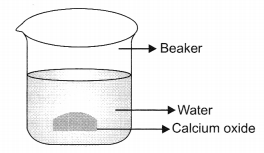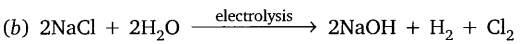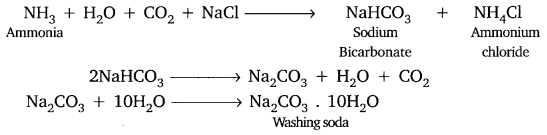# Acids, Bases and Salts Class 10 Important Questions Science Chapter 2

Solved the very best collection of Acids, Bases and Salts Class 10 Science Important Questions and Answers Chapter 2 Pdf from the latest NCERT edition books, It will help you in scoring more marks in CBSE Exams.

## Acids, Bases and Salts Class 10 Important Questions with Answers Science Chapter 2

### Acids, Bases and Salts Class 10 Important Questions Short Answer Type

Question 1.
(a) Give Arrhenius definition of an acid and a base.   (CBSE 2006)
(b) Choose a strong acid and strong base from the following:
CH3COOH, NH4OH, KOH, HCl
(a) Arrhenius definition for
Acid: Acids are those substances which release H+ ions when dissolved in water.
Base: Bases are those substances which release OH ions when dissolved in water,
(b) The strong acid is HCl and strong base is KOH.

Question 2.
(a) Write the formula and chemical name of bleaching powder.
(b) Write chemical equation to represent the action of atmospheric CO2 gas on bleaching powder when left exposed in open.
(c) State for what purpose is bleaching powder used in water treatment plants.   (CBSE 2006)
(a) CaOCl2 (Calcium oxychloride)(c) Bleaching powder is disinfectant, it can kill germs and hence, it is used in water treatment plant.

Question 3.
How will you test for the gas which is liberated when hydrochloric acid reacts with an active metal? (CBSE 2008/2012)
An active metal reacts with hydrochloric acid to give hydrogen gas. When a burning splinter is brought close to the gas evolved, pop sound is produced and the gas continues to burn.

Question 4.
What is ‘Baking Powder’? How does it make the cake soft and spongy?   (CBSE 2008)
Baking powder is a mixture of baking soda and tartaric acid.
When baking powder is heated it releases CO2 gas due to which the cake rises and becomes soft and spongy.

Question 5.
What happens chemically when quick lime is added to water?   (CBSE 2008)
Quick lime reacts with water to form calcium hydroxide (slaked lime) and large amount of heat is released (exothermic reaction) with a hissing sound.
CaO + H2O → Ca(OH)2 + heat

Question 6.
Name the gas evolved when dilute HCl reacts with sodium hydrogen carbonate. How is it recognised? (CBSE 2008)
The gas evolved is CO2.
It can be recognised by passing it through lime water (freshly prepared), the lime water turns milky.
Ca(OH)2 + CO2 → CaCO3

Question 7.
How does the flow of acid rainwater into a river make the survival of aquatic life in the river difficult?   (AI 2008)
Acid rain water when flows into the river the pH of river water decreases, which makes the aquatic life difficult to survive. The pH range suitable for aquatic survival is 7.0 to 7.8 and due to acid rainwater pH of river water decreases.

Question 8.
How is the pH of a solution of acid influenced when it is diluted?   (Foreign 2008)
Acid when dissolved in water the H+ concentration in moles per litre decreases and therefore pH will increase, as the solution becomes less acidic on dilution.

Question 9.
How does the pH of the solution change when a solution of the base is diluted?    (Foreign 2008)
Based on dilution with water become less basic in nature and their pH decreases.
(e.g. pH of the strong base would be 14, on diluting its pH becomes below 14)

Question 10.
Arrange the following in increasing order of their pH values:
NaOH soln, blood, lemon juice. (Foreign 2008)
Lemon juice < Blood < NaOH solution.

Question 11.
Two solutions A and B have pH values of 5 and 8 respectively. Which solution will be basic in nature?  (CBSE 2008C)
B will be basic nature, with pH = 8.

Question 12.
A compound ‘X’ of sodium is commonly used in kitchen for making crispy pakoras. It is also used for curing acidity in the stomach. Identify ‘X’. What is its chemical formula? State the reaction which takes place when it is heated during cooking.   (CBSE 2008C)
‘X’ is sodium hydrogen carbonate (baking soda)
Chemical formula → NaHCO3
During cooking → It decomposes to sodium carbonate, carbon dioxide and waterQuestion 13.
Fresh milk has a pH of 6. When it changes into curd (yoghurt) will it pH value increase or decrease? Why?  (CBSE 2009)
When fresh milk turns into curd, its pH value will decrease. This is because lactic acid is produced when milk changes into curd.

Question 14.
A compound which is prepared from gypsum has the property of hardening when mixed with a proper quantity of water. Identify the compound. Write the chemical equation for its preparation. For what purpose is it used in hospitals?     (CBSE 2009)
The compound is calcium sulphate hemihydrate, Plaster of Paris CaSO4. $$\frac{1}{2}$$H2O.It is used in hospitals for a plaster cast.

Question 15.
Why does tooth decay start when the pH of mouth is lower than 5.5?    (CBSE 2009)
When the pH in mouth is lower than 5.5, it means acids are formed due to food decay by bacteria in our mouth. The acid released reacts with enamel, which is made up of calcium phosphate and tooth decay starts.

Question 16.
What would be the colour of litmus in a solution of sodium carbonate?   (CBSE 2009)
Red litmus will change to blue colour in solution of sodium carbonate.

Question 17.
What effect does an increase in concentration of H+(aq) in a solution have on the pH of solution?   (AI 2009)
If the concentration of H+ ions in a solution increases, then the pH of solution decreases.

Question 18.
(i) Name the products obtained when sodium hydrogen carbonate is heated.
(ii) Write the chemical equation for the reaction involved in the above.   (AI 2009)
(i) When sodium hydrogen carbonate is heated. Sodium carbonate, carbon dioxide and water is formed.Question 19.
Which of these has a higher concentration of H+ ions – 1 M HCl or 1M CH3COOH?   (AI 2009)
1M HCl has higher concentration of H+ ions.

Question 20.
Crystals of copper sulphate are heated in a test tube for some time.
(a) What is the colour of copper sulphate crystals
(i) before heating and
(ii) after heating?    (AI 2009)
(b) What is the source of liquid droplets seen on inner side of tube?
(a) (i) Colour of copper sulphate crystals before heating is blue.
(ii) After heating blue colour disappears and turns white.
(b) It is the water of crystallisation.

Question 21.
Why does 1M HCl solution have a higher concentration of H+ ions than 1M CH3COOH solution?   (AI 2009)
HCl being a strong acid ionise completely to give more H+ ion than CH3COOH (a weak acid which ionise partially) of equal concentration.

Question 22.
Write the name and chemical formula of the calcium compound used for disinfecting drinking water. How is this compound manufactured?   (AI 2009)
Bleaching powder – CaOCl2
Preparation: On passing chlorine gas through slaked lime, CaOCl2 is formed.Question 23.
Which gas is generally liberated when a dilute solution of hydrochloric acid reacts with an active metal?   (Foreign 2009)
Hydrogen gas is liberated when a dilute solution of hydrochloric acid reacts with an active metal.
e.g. Zn(s) + 2HCl(dil) → ZnCl2(aq) + H2(g)

Question 24.
What is the colour of litmus in a solution of ammonium hydroxide?   (Foreign 2009)
Red litmus turns blue in solution of ammonium hydroxide.

Question 25.
What is an alkali?   (Foreign 2009)
Bases soluble in water are called alkali.

Question 26.
(a) Write the name given to bases that are highly soluble in water. Give an example,
(b) How is tooth decay related to pH? How can it be prevented?
(c) Why does bee sting cause pain and irritation? Rubbing of baking soda on the sting area gives relief. How?   (CBSE 2012)
(a) Alkali, e.g., NaOH (Sodium hydroxide).
(b) Lower the pH, more will be tooth decay. Acid reacts with Ca3(PO4)2 and cause tooth decay. It can be prevented by brushing teeth after every meal.
(c) It is due to formic acid. Sodium hydrogen carbonate (Baking soda) neutralises formic acid giving relief.

Question 27.
A white powder is added while baking breads and cakes to make them soft and fluffy. Write the name of the powder. Name its main ingredients. Explain the function of each ingredient. Write the chemical reaction taking place when the powder is heated during baking.   (CBSE 2012)
Baking powder. It consists of sodium hydrogen carbonate and tartaric acid. Sodium hydrogen carbonate on heating gives C02 which makes cake soft and fluffy. Tartaric acid neutralizes the bitterness due to sodium carbonate produced.Question 28.
“Sodium hydrogen carbonate is a basic salt”. Justify the statement. How is it converted into washing soda? Explain. (CBSE 2012)
Sodium hydrogen carbonate is a salt of sodium hydroxide (strong base) and carbonic acid (weak acid).
Thus, it is a basic salt. It is converted into washing soda by heating followed by crystallization.Question 29.
A student detected the pH of four unknown solution A, B, C and D as follows 11, 5, 7 and 2. Predict the nature of the solution.   (CBSE 2013)
A is basic ‘B’ is acidic ‘C’ is natural and ‘D’ is strongly acidic.

Question 30.
(i) Give the constituents of baking powder.
(ii) Why cake or bread swells on adding baking powder? Write chemical equation.    (CBSE 2013)
(i) Baking powder contain sodium hydrogen carbonate and tartaric acid.
(ii) It is due to carbon dioxide
$$2 \mathrm{NaHCO}_{3}(\mathrm{s}) \stackrel{\text { heat }}{\longrightarrow} \mathrm{Na}_{2} \mathrm{CO}_{3}(\mathrm{s})+\mathrm{CO}_{2}(\mathrm{g})+\mathrm{H}_{2} \mathrm{O}(l)$$

Question 31.
Equal length of magnesium ribbon are taken in two test tubes A’ and ‘B’, H2SO4 is added to test tube ‘A’ and H2CO3 in the test tube ‘B’ in equal amounts:       (CBSE 2013)
(a) Identify the test tube showing vigorous reaction.
(c) Name the gas liberated in both the tubes. How will you prove its liberation?
(d) Write chemical equations for both reactions.
(e) Out of the two acids taken above
(i) which one will have lower pH value.
(ii) lower H+ concentration respectively.
(a) A will show vigorous reaction.
(b) It is because H2SO4 is strong acid.
(c) Hydrogen gas will be formed. Bring a burning splinter near the gas. It will burn with ‘pop’ sound. It shows gas liberated is hydrogen.
(d) Mg + H2SO4 → MgSO4 + H2
Mg + H2CO3 → MgCO3 + H2
(e) ‘A’ (H2SO4) will have lower pH.
‘B’ (H2CO3) will have lower concentration of H+

Question 32.
Name the natural source of each of the following acid.
(i) Citric acid.
(ii) Oxalic acid.
(iii) Lactic acid.
(iv) Tartaric acid.     (CBSE 2014)
(i) Lemon and orange
(ii) Tomatoes and Guava.
(iii) Sour milk (curd)
(iv) Tamarind.

Question 33.
Explain the action of dilute hydrochloric acid on the following with chemical equation:
(i) Magnesium ribbon
(ii) Sodium hydroxide
(iii) Crushed egg shells.    (CBSE 2015)
(i) Hydrogen gas will be formed
Mg(s) + 2HCl (dil) → MgCl2(aq) + H2(s)
(ii) Sodium chloride and water will be formed
NaOH + HCl → NaCl + H2O
(iii) Crushed egg shell are made up of CaCO3 which reacts with dil HCl to give brisk
effervescence due to CO2.
CaCO3 + 2HCl → CaCl2 + H2O + CO2

Question 34.
Name the acid present in the following:
(i) Tomato
(ii) Vinegar
(iii) Tamarind   (CBSE 2015)
(i) Oxalic acid
(ii) Acetic acid
(iii) Tartaric acid

Question 35.
15 ml of water and 10 ml of sulphuric acid are to be mixed in a beaker
(i) State the method that should be followed with reason.
(ii) What is this process called?    (CBSE 2015)
(i) The acid is to be added slowly in water to prevent the mixture to be splashed.
The reaction is highly exothermic, therefore, constant cooling should be done
(ii) The process is called dilution.

Question 36.
(a) Define olfactory indicators. Name two substances which can be used as olfactory indicator.
(b) Choose strong acids from the following:
CH3COOH, H2SO4, H2CO3, HNO3       (CBSE 2015)
(a) Those substances whose smell (odour) changes in acidic or basic solution are called olfactory indicators, e.g., onion and vanilla.
(b) H2SO4 and HNO3 are strong acids.

Question 37.
A white coloured powder is used by doctors for supporting fractured bones.
(a) Write chemical name and formula of the powder
(b) When this white powder is mixed with water a hard solid mass is obtained.
Write balanced chemical equation for the change.      (CBSE 2015)### Acids, Bases and Salts Class 10 Important Questions Long Answer Type

Question 1.
What is meant by water of crystallisation in a substance? How would you show that blue copper sulphate crystals contain water of crystallisation?     (CBSE 2008)
Water of crystallization is fixed number of water molecules present in the crystalline state of salt.
e.g., CUSO4.5H2O Copper sulphate [Blue vitriol]
FeSO4.7H2O Ferrous sulphate [Green vitriol]

To show the water of crystallisation in copper sulphate:
Take a dry boiling test tube, add few crystals of blue copper sulphate and heat it on the flame. Water vapour collects on the inner sides of the test tube. The colour of copper sulphate changes to white.

On cooling add few drops of water to anhydrous copper sulphate (white powder obtained) it turns blue.

Question 2.
Write the chemical formula for washing soda. How may it be obtained from baking soda? Name an industrial use of washing soda other than washing clothes.     (AI 2008)
Washing soda → Na2CO3 + 10H2O. (sodium carbonate decahydrate)
Baking soda on heating produces sodium carbonate which is then recrystallised.
2NaHCO3 → Na2CO3 + CO2 + H2O
Na2CO3 + 10H2O → Na2CO3.10H2O
It is used in the manufacture of glass and paper.

Question 3.
Write the chemical formula of bleaching powder, how is bleaching powder prepared?
For what purpose is it used in paper factories?     (AI 2008)
Bleaching powder – CaOCl2
Preparation: On passing chlorine gas over slaked lime (Ca(OH)2), bleaching powder is obtained.
Ca(OH)2 + Cl2 → CaOCl2 + H2O
Use: It is used in paper industry for bleaching paper.

Question 4.
Write the chemical formula of washing soda and baking soda. Which one of these two is an ingredient of antacids? How does it provide relief in stomach ache?       (Foreign 2008)
Washing soda – Na2CO3.10H2O (Sodium carbonate decahydrate)
Baking soda – NaHCO3 (Sodium bicarb3onate)
Baking soda is used as an antacid it neutralises excess acid in the stomach and provides relief.

Question 5.
Solid calcium oxide was taken in a container and water was added slowly to it.
(i) State the two observations made in the experiment.
(ii) Write the name and chemical formula of the product formed.    (AI 2008C)(i) A large amount of heat is evolved → Exothermic reaction
The reaction takes place vigorously with hissing noise and bubbles.
(ii) Ca(OH)2   (Calcium hydroxide), common name is slaked lime.

Question 6.
(a) Why does an aqueous solution of an acid conduct electricity?
(b) How does the concentration of hydronium ions (H3O+) change when a solution of an acid is diluted?
(c) Which has higher pH value, a concentrated or dilute solution of hydrochloric acid?
(d) What do you observe on adding dilute hydrochloric acid to
(i) Sodium carbonate placed in test tube.
(ii) Zinc metal in a test tube?    (AI 2008C)
(a) Aqueous solution of an acid releases ions so that it can conduct electricity.
(b) When solution of an acid is diluted, concentration of hydronium ions (H3O+) decreases.
(c) Dilute solution of HCl will have higher pH than concentrated solution.
(d) (i) Na2CO3 + 2HCl(dil.) → 2NaCl + CO2 + H2O
Effervescence of CO2 gas is seen.
(ii) Zn(s) + 2HCl(dil.) → ZnCl2 + H2(g)
Odourless and colourless gas hydrogen is evolved with bubbles on surface of zinc.

Question 7.
What is meant by ‘water of crystallisation’ of a substance? Describe an activity to show that blue copper sulphate crystals contain water of crystallisation.     (Foreign 2009)
The water molecules associated with a crystalline substance is called ‘water of crystallisation’.
Activity → Take few crystals of blue copper sulphate in a dry boiling tube. Heat it
on flame, the blue crystals change to white dry powder and the inner surface of the test tube condenses with water.
This proves that crystals contain water molecules in it. If we add some water in the white powder it regains the lost water molecules and become blue again.

Question 8.
(a) Identify the acid and the base whose combination forms the common salt that you use in your food. Write its formula and chemical name of this salt. Name the source from where it is obtained.
(b) What is rock salt? Mention its colour and the reason due to which it has this colour.
(c) What happens when electricity is passed through brine? Write the chemical
equation for it.        (CBSE 2012)
(a) HCl is acid and NaOH is base whose combination forms the common salt. Its formula is NaCl (Sodium chloride). It is obtained from seawater.
(b) Rock salt is the common name for the mineral “halite”. Its chemical formula is NaCl. It may be white or light blue or yellow depending upon impurities present
in it.
(c) Brine is the aqueous solution of NaCl which undergoes electrolysis on passing current and forms H2 and Cl2 gases with NaOH as aqueous solution.
$$2 \mathrm{NaCl}+2 \mathrm{H}_{2} \mathrm{O} \stackrel{\text { electrolysis }}{\longrightarrow} 2 \mathrm{NaOH}+\mathrm{H}_{2}+\mathrm{Cl}_{2}$$

Question 9.
(i) Explain why is hydrochloric acid a strong acid and acetic acid, a weak acid. How can it be verified?
(ii) Explain why aqueous solution of an acid conducts electricity.
(iii) You have four solutions A, B, C and D. The pH of solution A is 6, B is 9, C is 12 and D is 7.
(a) Identify the most acidic and most basic solutions.
(b) Arrange the above four solutions in the increasing order of H+ ion concentration.
(c) State the change in colour of pH paper on dipping in solution C and D.      (CBSE 2012)
(i) HCl is completely ionised in aqueous solution whereas acetic acid is partially ionised in aqueous solution. HCl gives dark red colour with pH paper whereas CH3COOH gives orange colour.
(ii) It is because acid ionises in aqueous solution and these ions conduct electricity.
(iii) (a) ‘A’ is most acidic and ‘C’ is most basic.
(b) C(10-12) < B (10-9) < D(10-7) < A(10-6)
(c) pH paper will become blue in ‘C’ and green in ‘D’.

Question 10.
(i) Dry pellets of a base ‘X’ when kept in open absorbs moisture and turns sticky.
The compound is also formed by chlor-alkali process. Write chemical name and formula of X. Describe chlor-alkali process with balanced chemical equation. Name the type of reaction occurs when X is treated with dilute hydrochloric acid. Write the chemical equation.
(ii) While diluting an acid, why is it recommended that the acid should be added to water and not water to the acid? (CBSE 2012)
(i) X is sodium hydroxide, NaOH.
When sodium chloride solution (brine solution) is electrolysed, sodium hyroxide solution is formed. H2 and Cl2 gases are liberated. This is chlor-alkali process.The above reaction is neutralization reaction.
(ii) It is because process is highly exothermic. If water is added to acid, it generates huge amount of heat which may cause accident but if acid is added dropwise to water, then the heat is absorbed by the solution.

Question 11.
A student dropped few pieces of marble in dilute hydrochloric acid, contained in a test-tube. The evolved gas was then passed through lime water. What change would be observed in lime water? What will happen if excess of gas is passed through lime water? With the help of balanced chemical equations for all the changes explain the observations.   (CBSE 2012)
CaCO3 + 2HCl (dil.) → CaCl2 + H2O + CO2
Lime water will turn milky due to liberation of CO2.
Ca(OH)2(aq) + CO2(g) → CaCO2(s) + H2O(l)
If excess of CO2 gas is passed through lime water, milkiness will disappear due to the formation of Ca(HCO3)2(aq) which is soluble in water.
CaCO3(s) + H2O(l) + CO2(g) → Ca(HCO3)2(aq)

Question 12.
(a) Identify the compound of calcium which is yellowish-white powder and is used for disinfecting drinking water. Write its chemical name and formula. How is it manufactured? Write the chemical equation for the reaction involved. Also list two other uses of the compound.
(b) Write the balanced chemical equation of Chlor-alkali process.    (CBSE 2012)
(a) The compound is bleaching powder (CaOCl2). Its chemical name is calcium oxychloride. It is manufactured by reaction of solid slaked lime with dry chlorine gas.
Ca(OH)2 + Cl2 → CaOCl2 + H2O
(i) It is used for bleaching purposes in laundary, textile and paper industries.
(ii) It is used for preparation of chloroform.Question 13.
(a) Crystals of a substance changed their colour on heating in a closed test tube but regained it after sometime when they were allowed to cool down. Name the substance and write its formula and explain the phenomenon involved.
(b) Name the compound whose one formula unit is associated with 10 water molecules. How is it prepared? Give equations of related reactions. Give two uses of the compound.      (CBSE 2012)It regains its colour by absorbing water from atmosphere and becomes blue in colour.
CuSO4 + 5H2O → CuSO4.5H2O
(b) Na2CO3.10H2O. It is called sodium carbonate decahydrate or washing soda. It is prepared by passing CO2 gas through saturated solution of ammonical brine.Uses:
(i) It is used in the production of washing powder.
(ii) It is used for the manufacture of glass.

Question 14.
(a) A metal compound ‘X’ reacts with dil. H2SO4 to produce effervescence. The gas evolved extinguishes a burning candle. If one of the compound formed is calcium sulphate, then what is ‘X’ and the gas evolved? Also, write a balanced chemical equation for the reaction which occurred.
(b) (i) Name one antacid. How does it help to relieve indigestion in stomach?
(ii) A farmer treats the soil with quicklime or calcium carbonate. What is the nature of soil? Why does the farmer treat the soil with quicklime? (CBSE 2012)
(a) ‘X’ is CaCO3 (calcium carbonate). The gas evolved is CO2.
CaCO3 + H2SO4 (dil.) → CaSO4 + H2O + CO2
(b) (i) NaHCO3 is antacid. It neutralizes excess of acid formed in the stomach.
(ii) The soil is acidic in nature. The farmer wants to make it neutral by adding quicklime which neutralise the acid in the soil.

Question 15.
(a) State the chemical properties on which the following uses of baking soda are based:
(i) as an antacid
(ii) as a soda acid fire extinguisher
(iii) to make bread and cake soft and spongy.
(b) How is washing soda is obtained from baking soda? Write balanced chemical equation.     (CBSE 2015)
(a) (i) It is weakly basic in nature and naturalize hyperacidity
(ii) It liberates CO2 with H2SO4, which extinguish fire.
(iii) It liberates CO2 on heating which makes bread and cake soft and spongy.
(b) $$2 \mathrm{NaHCO}_{3} \stackrel{\text { heat }}{\longrightarrow} \mathrm{Na}_{2} \mathrm{CO}_{3}+\mathrm{CO}_{2}+\mathrm{H}_{2} \mathrm{O}$$
Baking soda on heating gives sodium carbonate which on crystallisation forms washing soda
Na2CO3 + 10H2O → Na2CO3.10H2O.

Question 16.
State reason for the following statements:
(i) Tap water conducts electricity whereas distilled water does not.
(ii) Dry hydrogen chloride gas does not turn blue litmus red whereas dilute hydrochloric acid does.
(iii) During summer season, a milk man usually adds a very small amount of baking soda to fresh milk.
(iv) For a dilution of acid, acid is added into water and not water into acid.
(v) Ammonia is a base but does not contain hydroxyl group.      (CBSE 2015)